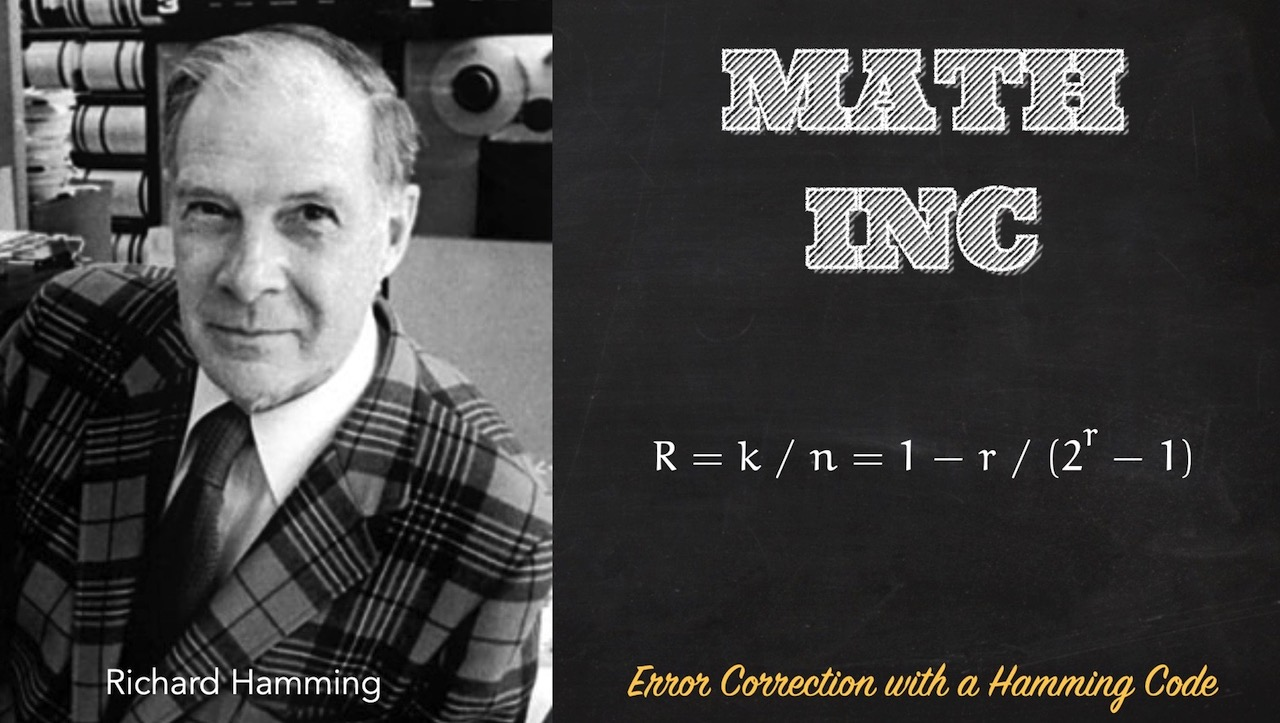👋🏼

# Error Correction, Part 2

This video contains and algorithm that you could very well be asked about in an interview: The Hamming Code. It's a deceptively simple algorithm to understand, very difficult to implement (at least for me).

We can use this algorithm to detect whether an error occurred in a digital transmission of any size - knowing precisely where it is and how to correct it.

It's a fun puzzler and I'll show you my solution in this video.

## The Code

Here's the code used in the video. There's always room for improvement - feel free to suggest! Your comments, as always, are welcome and you can drop me an email or, when the code is published, feel free to leave an issue on GitHub.

Here are our utility functions:

``````const getParityBitPositions = (message) => {
let matrix={};
for(let i=1; i <= message.length; i = i * 2) {
let taken=0; skipped=0;
matrix[i] = [];
for(let x = i; x <= message.length; x++){
if(taken < i) {
matrix[i].push(x)
taken+=1;
}else{
skipped+=1;
}
if(skipped===taken) skipped = taken = 0;
}
}
return matrix;
}

const digits= message.split('');
const matrix = getParityBitPositions(message)
//add parity bits "_" to positions that are powers of two
//as parity bits. (positions 1, 2, 4, 8, 16, 32, 64, etc.)
for(let b of Object.keys(matrix)){
//...
digits.splice(b-1, 0, "_")
}
return digits.join("");
}

const flipABit = function(message,n){
const binaryMessage = message.split("");
console.log("Flipping at",n);
binaryMessage[n] = binaryMessage[n] === "1" ? "0" : "1";
return binaryMessage.join("")
}
``````

Next up, our parity calculators/correctors. Note that you'll need to export both of these if you want to plug them into your encoder.

``````exports.calcParity = (message) => {
const matrix = getParityBitPositions(withPlaceholders);
const binaryMessage = withPlaceholders.split('');

for(let bitIndex of Object.keys(matrix)){
let thisCalc=0;
for(let idx of matrix[bitIndex]){
if(binaryMessage[idx-1] === "1") thisCalc+=1;
}
// if(thisCalc % 2 > 0) console.log(`Setting B\${bitIndex} to 1 because \${thisCalc}`)
// else console.log(`Leaving B\${bitIndex} as 0 because \${thisCalc}`);
binaryMessage[bitIndex - 1] = thisCalc % 2 > 0 ?  "1" : "0"
}
return binaryMessage.join("")
}

for(let idx of Object.keys(matrix)){
let dataBits = matrix[idx], dataBitSum = 0;
for(let bit of dataBits){
const bitIdx = parseInt(bit) - 1; //no off by 1!
dataBitSum+= parseInt(binaryMessage[bitIdx]);
}
if(dataBitSum % 2 !== 0) { //the error check
console.log("Found error with Parity bit",idx);
}
}
if(errorPosition > 0){
//flip the error using the array of chars
console.log("Correcting error at position",errorPosition-1);
binaryMessage[errorPosition-1] = binaryMessage[errorPosition-1] === "1" ? "0" : "1";
}
return binaryMessage.join("");
}

exports.removeParityBits = (message) => {
const digits= message.split('');
const matrix = getParityBitPositions(message);
const bitPositions = Object.keys(matrix);
//gotta go in reverse here because the index positions will change as we mutate the array
for(let i = bitPositions.length-1; i >=0; i--){
const bitPosition = bitPositions[i];
digits.splice(bitPosition-1, 1);
}
return digits.join("");
}
``````